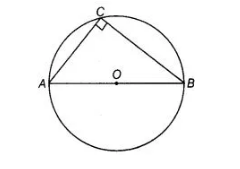# If AOB is a diameter of a circle`
Question:

If AOB is a diameter of a circle and C is a point on the circle, then AC2+ BC2 = AB2.

Solution:

True

Since, any diameter of the circle subtends a right angle to any point on the circle.

If AOB is a diameter of a circle and C is a point on the circle, then ΔACB is right angled at C. In right angled ΔACB, [use Pythagoras theorem]

AC2 + BC2 = AB2